Isoperimetrical Polyiamonds

The following tables show (a) the numbers of polyominoes of a given area, A, and perimeter, P, and (b) the total areas of these sets of pieces. These tables were supplied by Brendan Owen.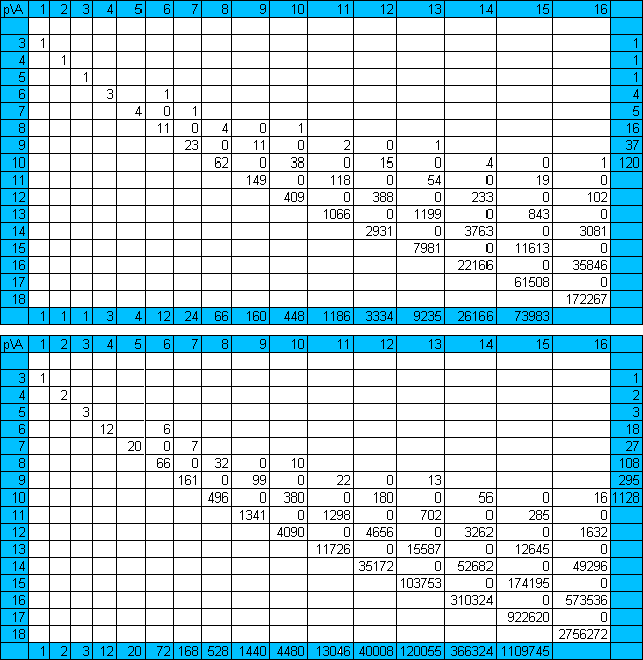If we consider complete sets of a particular perimeter then with perimeters 6 and 7 there are, respectively, 4 and 5 pieces.With perimeter 8 there are 16 pieces covering a total area of 108 unit triangles. Unlike the isoperimetric polyominoes the areas of isoperimetric polyiamonds are even when the peimeter is even and odd when the perimeter is odd. This gives us parity problems with the even perimeter pieces and any construction made with this set must have a colouring excess of 2 or 6. Four examples are shown below. The figure at the top right is by Livio Zucca and the one at the bottom right is by Patrick Hamlyn.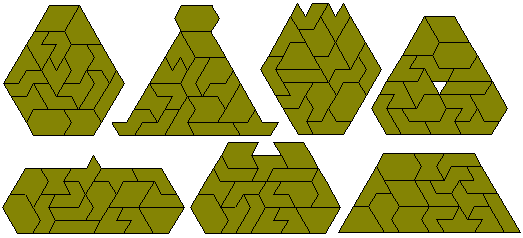The one-sided set consists of 24 pieces covering an area of 158 triangles.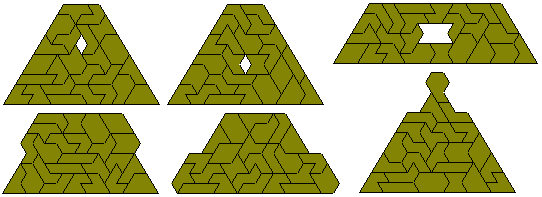There are 37 polyiamonds with perimeter 9 covering a total area of 295.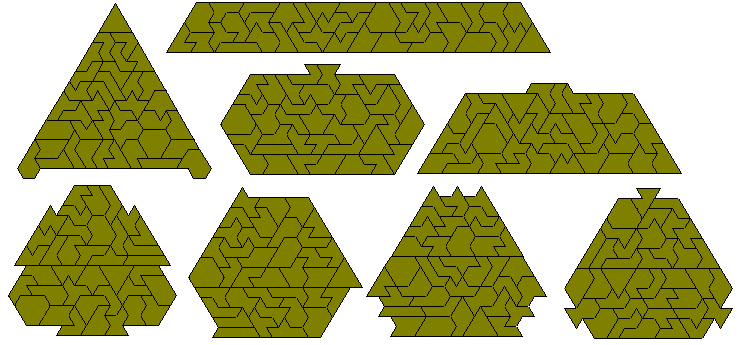It is also possible to make the two triangles below with the full set..Brendan Owen has found the 120 perimeter 10 polyiamonds. This set covers an area of 1128 unit triangles which can form 6x94 and 12x47 parallelograms. A 41-12 trapezium is also possible. Since each piece has an even area the maximum nuber of congruent pieces possible would be twelve. Constructions with six and twelve congruent figures are shown below.Patrick Hamlyn has made the following construction with the set. This is the maximum number of all different sized triangles which can be made using the entire set. It is possible to make more triangles using the whole set, and probably also more all different sized triangles if you allow pieces to be omitted. It may even be possible to make more different sized triangles using the whole set if you allow more than one triangle of one of the sizes.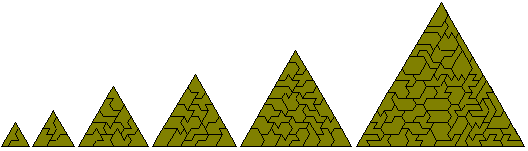It is also possible to make multiple regular hexagons with this set.